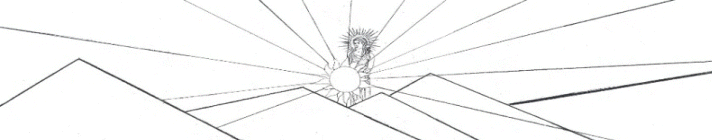Guitar Outline Equation y = (A*sin(B*x+C) + D*sin(E*x+F) + G*sin(H*x+I) + J*sin(K*x+L)) * (M + N*x + O*x^2 +P*x^3 + Q*x^4) * atan(R*x) * atan(S*(BL-x))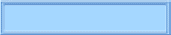The Nealon Equation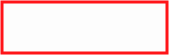The GuitarsThe Arch TopsThe Classicals webpages and Eos image copyright 2015   M  NealonProof of Equations 3 - 6, or How To Draw an Inside Curve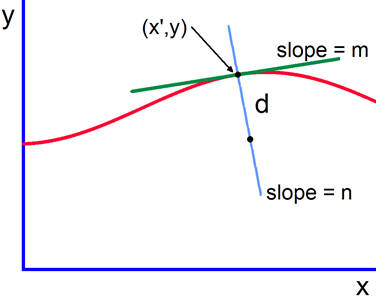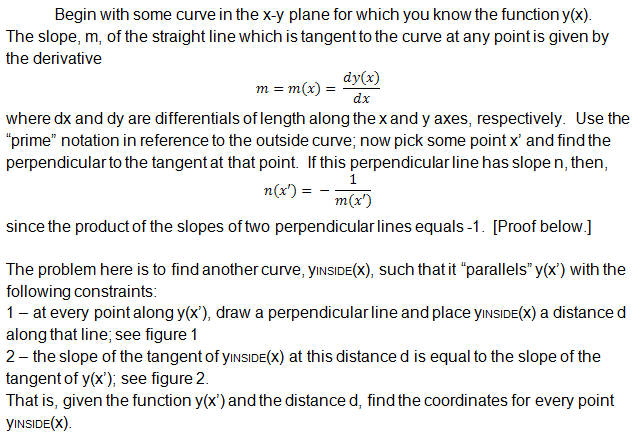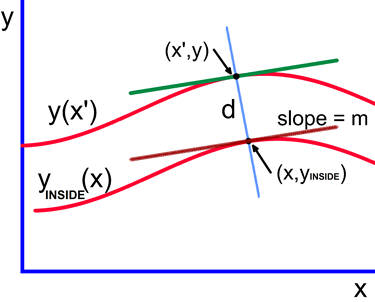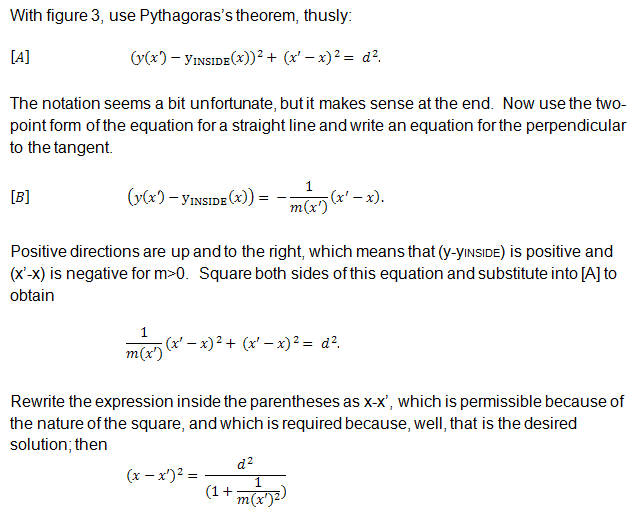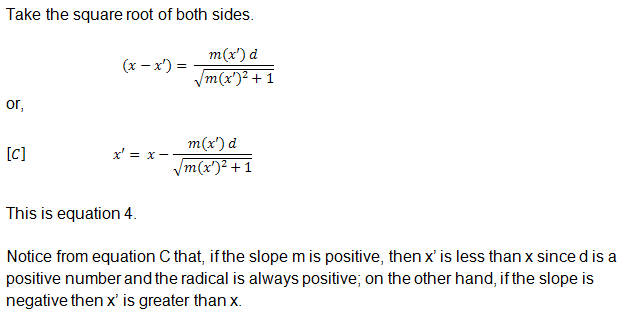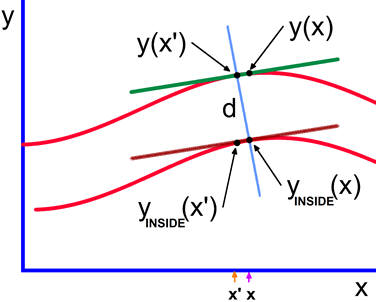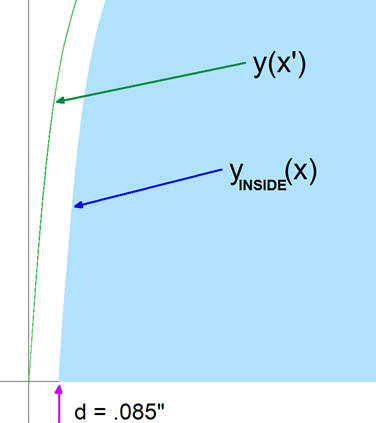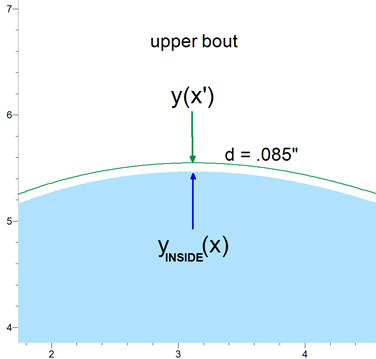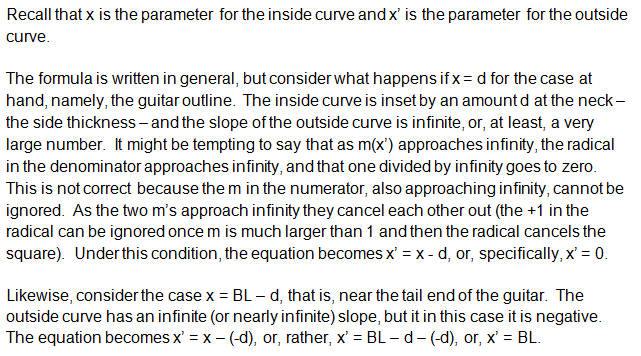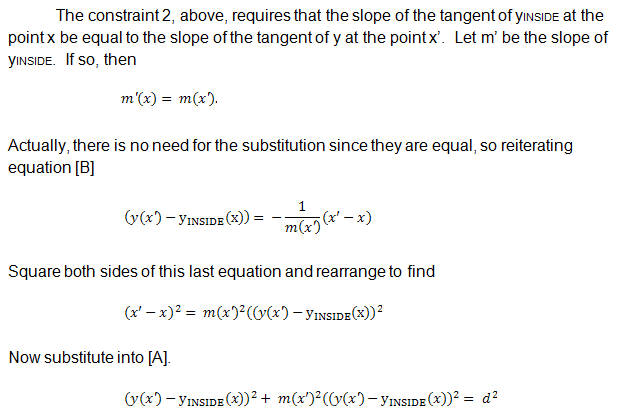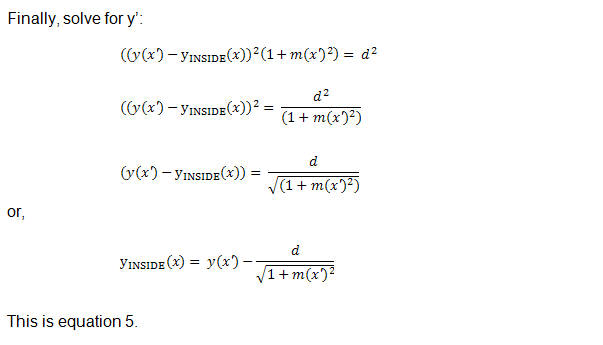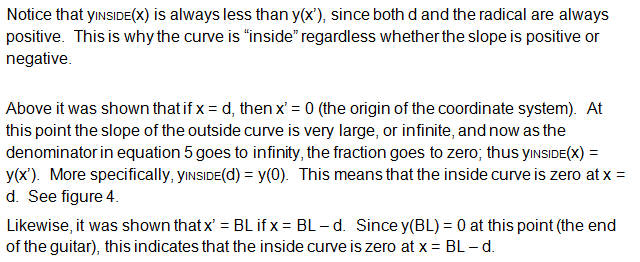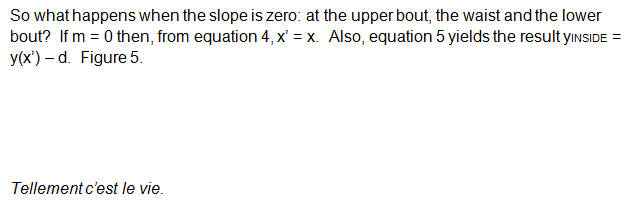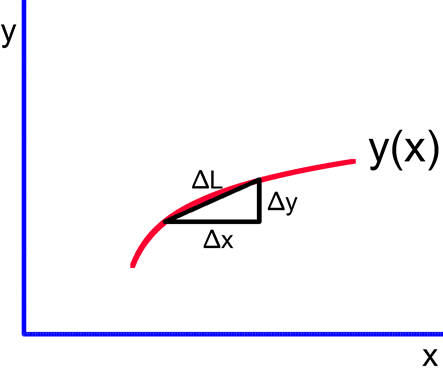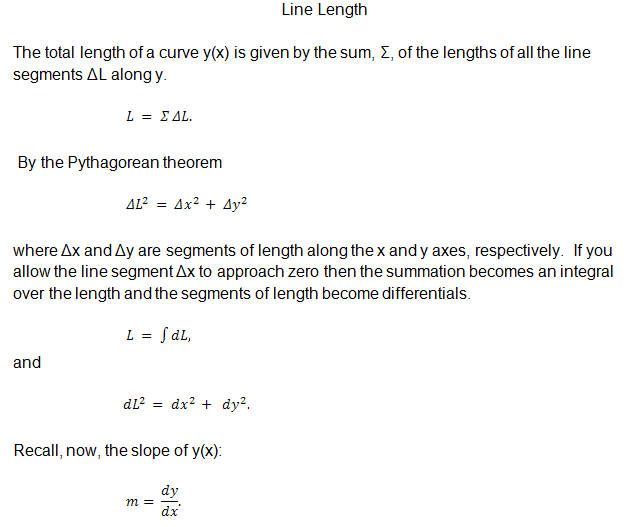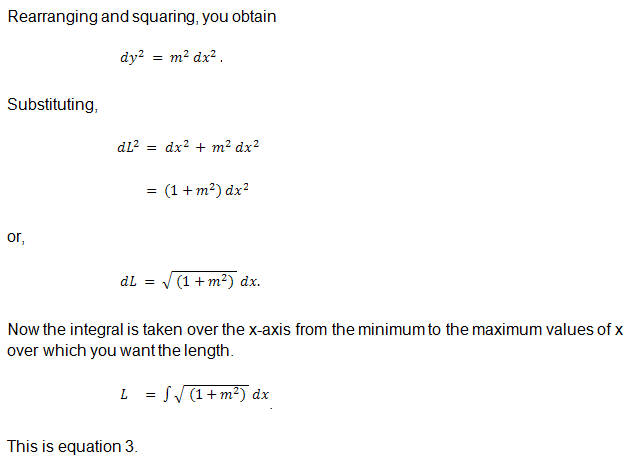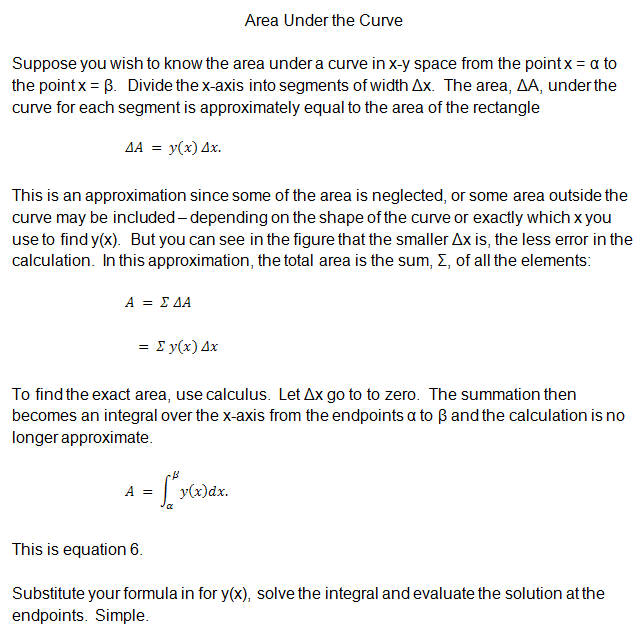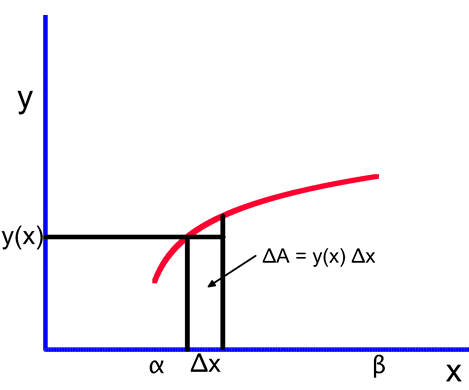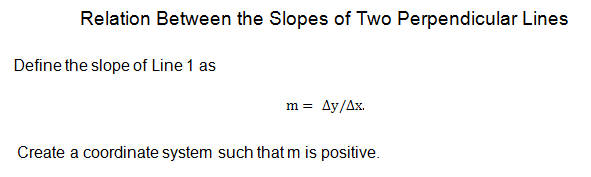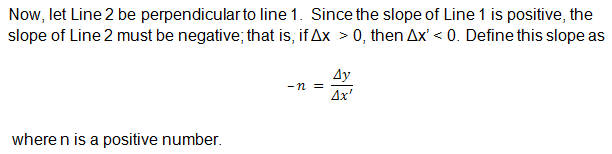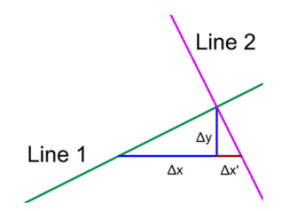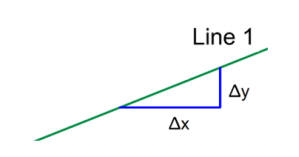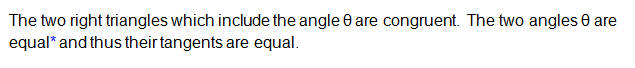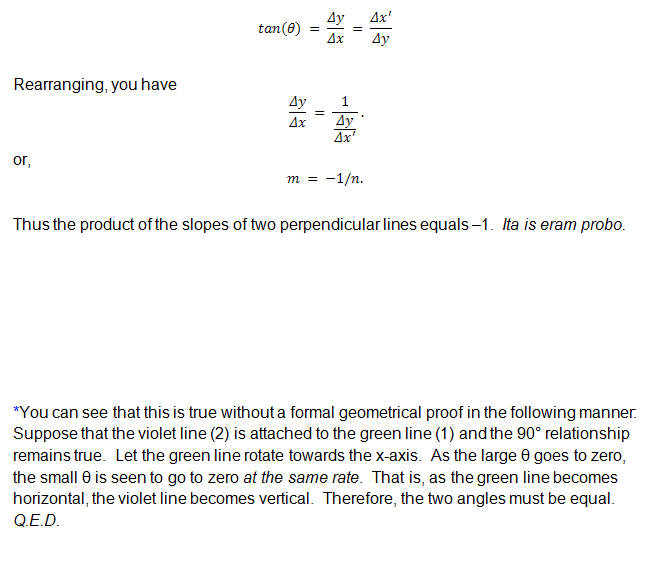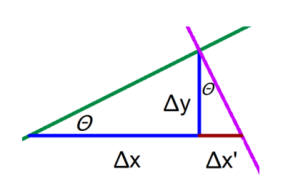aaaaaaaaaaaaiii